# SAO Buffs/Magic Skills BreakdownBreakdown of Magic/Buff Skills for Sword Art Online Characters

This is a list of all the magic that SAO characters get. AW characters are all unique so If you want information on them, you will need to look at their specific character pages.

For the list of SAO charas Sword Skills & Weapon Breakdowns:Note That All the information on this page was taken from the Strategy Guide which is riddled with errors. I tried to correct a lot of the errors but I’m still not 100% sure about everything on this page. If you find out something that isn’t correct here, tell me.

### ~BUFFS & DEBUFFS~

#### SKILL DESCRIPTIONVenom Shot  Fire small venom ball. Low chance to poison enemy. Poison Lasts 45 Seconds and Drains 50% HP. Power increases with skill level.

• MP Cost:
• Lv1 & over = 200MP
• Lv200 & over = 250MP
• Lv300 & over = 300MP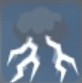Thunderbolt  Fire small thunder ball. Low chance to paralyze enemy. Paralysis Lasts 10 Seconds. Power increases with skill level.

• MP Cost:
• Lv1 & over = 200MP
• Lv200 & over = 250MP
• Lv300 & over = 300MPBattle Shout  Draw aggro from enemies within range.*No effect during online play.

• MP Cost: 350MP
• Range:
• Lv1= 10m
• Lv500 = 15m
• Lv1000 = 30m
• Hate Value:
• Lv1 = 4000
• Lv500 = 18000
• Lv1000 = 36000Bind  Inflict Bind status on enemy. Cast time is reduced with skill level.

• MP Cost:
• Lv1 & over = 500MP
• Lv200 & over = 600MP
• Lv300 & over = 750MPMagia  Increases Party Magical Power by 30% (Includes healing magic).

• MP Cost:
• Lv1 & over = 300MP
• Lv200 & over = 350MP
• Lv300 & over = 400MP
• Duration:
• Lv1 = 60 Seconds
• Lv500 = 90 Seconds
• Lv1000 = 180 SecondsQuick  Increases Party Movement Speed by 50%.

• MP Cost:
• Lv1 & over = 300MP
• Lv200 & over = 350MP
• Lv300 & over = 400MP
• Duration:
• Lv1 = 60 Seconds
• Lv500 = 90 Seconds
• Lv1000 = 180 SecondsSharpness  Increases Party Attack by 20%.

• MP Cost:
• Lv1 & over = 300MP
• Lv200 & over = 350MP
• Lv300 & over = 400MP
• Duration:
• Lv1 = 60 Seconds
• Lv500 = 90 Seconds
• Lv1000 = 180 SecondsProtection  Increases Party Defense by 17%.

• MP Cost:
• Lv1 & over = 300MP
• Lv200 & over = 350MP
• Lv300 & over = 400MP
• Duration:
• Lv1 = 60 Seconds
• Lv500 = 90 Seconds
• Lv1000 = 180 SecondsExhaust  Decreases Enemy Attack by 20%.

• MP Cost:
• Lv1 & over = 300MP
• Lv200 & over = 350MP
• Lv300 & over = 400MP
• Duration:
• Lv1 = 60 Seconds
• Lv500 = 90 Seconds
• Lv1000 = 180 SecondsWeakness  Decreases Enemy Defense by 25%.

• MP Cost:
• Lv1 & over = 300MP
• Lv200 & over = 350MP
• Lv300 & over = 400MP
• Duration:
• Lv1 = 60 Seconds
• Lv500 = 90 Seconds
• Lv1000 = 180 SecondsSlow  Decrease Enemy Movement Speed by 50%.

• MP Cost:
• Lv1 & over = 300MP
• Lv200 & over = 350MP
• Lv300 & over = 400MP
• Duration:
• Lv1 = 60 Seconds
• Lv500 = 90 Seconds
• Lv1000 = 180 SecondsHiding  Become semi-invisible. Enemies can still see you, but aggro rate is decreased by 50%. Duration increases with skill level.*Has no effect in online play.

• MP Cost: 400MP
• Duration:
• Lv1 = 30 Seconds
• Lv500 = 45 Seconds
• Lv1000 = 90 SecondsHollow Body  Become fully invisible. Unable to be seen on the mini-map or locked onto. Any action other than movement cancels the invisibility. Duration increases with skill level.

• MP Cost: 400MP
• Duration:
• Lv1 = 30 Seconds
• Lv500 = 45 Seconds
• Lv1000 = 90 SecondsSearcher  Allows player to see all treasure & Sudden Quests on the map. The Depth of The Color of the Sudden Quest on the map dictates its height.

• MP Cost: 100MP
• Duration:
• Lv1 = 30 Seconds
• Lv500 = 45 Seconds
• Lv1000 = 90 Seconds

### ~HEALING~

Keep in mind that the value recovered by healing is affected by the difficulty you are playing. I didn’t know whether I should present the Online Mode values or the Normal Mode Offline values that are listed in the Strategy Guide so I decided to just do the normal values given in the Strategy Guide.

Difficulty Multipliers:

• Easy Mode: Recovery multiplied by 2
• Normal Mode: Standard Values
• Hard Mode: Recovery divided by 2
• Online Mode: Very sure its the same as hard mode.

#### SKILL DESCRIPTIONFirst Aid  Recover self HP.

• MP:
• Lv1 & over = 150MP
• Lv200 & over = 160MP
• Lv300 & over = 200MP
• Recovery Amount:
• Lv1 = 20%
• Lv500 = 35%
• Lv1000 = 50%Heal  Recover Party HP.

• MP:
• Lv1 & over = 450MP
• Lv200 & over = 500MP
• Lv300 & over = 650MP
• Recovery Amount:
• Lv1 = 20%
• Lv500 = 35%
• Lv1000 = 50%Refresh  Greatly Recover Party HP.

• MP:
• Lv1 & over = 1500MP
• Lv200 & over = 1600MP
• Lv300 & over = 2000MP
• Recovery Amount:
• Lv1 = 50%
• Lv500 = 75%
• Lv1000 = 100%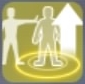Resurrection  Brings allies back from dead (Within Range).

• MP:
• Lv1 & over = 800MP
• Lv200 & over = 850MP
• Lv300 & over = 1000MP
• Recovery Amount:
• Lv1 = 50%
• Lv500 = 75%
• Lv1000 = 100%Cure  Recovers Party Status ailments.*Unable to cure stat debuffs

• Number of Ailments Recovered:
• Lv1 = 1
• Lv500 = 4
• Lv1000 = 9
• MP:
• Lv1 & over = 150MP
• Lv200 & over = 160MP
• Lv300 & over = 200MP
• Range:
• Lv1 = Self
• Lv500 = 10m
• Lv1000 = 20mRegenerate   Slowly regenerates player HP over a period of time.

• Duration: 120 Seconds
• MP:
• Lv1 & over = 150MP
• Lv200 & over = 160MP
• Lv300 & over = 200MP
• HP Recovery over Duration:
• Lv1 = 30%
• Lv500 = 66%
• Lv1000 = 102%Mana Regenerate  Increases rate of player MP regeneration.

• Duration: 120 Seconds
• MP:
• Lv1 & over = 20MP
• Lv200 & over = 250MP
• Lv300 & over = 400MP
• MP Recovery over Duration:
• Lv1 = 10%
• Lv500 = 20%
• Lv1000 = 30%Stamina Boost  Decreases amount of Stamina used.

• Duration: 120 Seconds
• MP:
• Lv1 & over = 150MP
• Lv200 & over = 160MP
• Lv300 & over = 200MP
• SP Cost Decrease:
• Lv1 = 15%
• Lv500 = 21%
• Lv1000 = 30%Revive  Allows the user to come back from the dead. One use per cast.

• Duration: 180 Seconds
• Recovery Amount:
• Lv1 = 20%
• Lv500 = 35%
• Lv1000 = 50%
• MP:
• Lv1 & over = 800MP
• Lv200 & over = 850MP
• Lv300 & over = 1000MP

### ~FIRE~

#### SKILL DESCRIPTION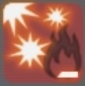Fireball  Fires a small fireball at an enemy.

• MP Cost:
• Lv1 & over = 60MP
• Lv200 & over = 70MP
• Lv300 & over = 100MP
• Number of Shots:
• Lv1 = 5
• Lv500 = 18
• Lv1000 = 30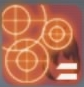Flame Blast  Drops a large fireball upon an enemy.

• MP Cost:
• Lv1 & over = 250MP
• Lv200 & over = 300MP
• Lv300 & over = 400MP
• Number of Shots:
• Lv1 = 3
• Lv500 = 6
• Lv1000 = 9Meteor Explosion  Summon a meteor from the sky to drop on an area surrounding target.

• MP Cost:
• Lv1 & over = 1600MP
• Lv200 & over = 1850MP
• Lv300 & over = 2500MPResist Fire  Increases Party Fire Resistance by 33%.

• MP Cost:
• Lv1 & over = 200MP
• Lv200 & over = 220MP
• LV300 & over = 250MP
• Duration:
• Lv1 = 60 Seconds
• LV500 = 90 Seconds
• LV1000 = 180 SecondsEnhance Fire  Increases Party Fire Based Damage by 30%.

• MP Cost:
• Lv1 & over = 300MP
• Lv200 & over = 350MP
• Lv300 & over = 400MP
• Duration:
• Lv1 = 60 Seconds
• Lv500 = 90 Seconds
• Lv1000 = 180 Seconds

### ~ICE~

#### SKILL DESCRIPTION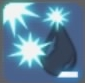Ice Bullet  Fires a small icicle at an enemy.

• MP Cost:
• Lv1 & over = 80MP
• Lv200 & over = 100MP
• Lv300 & over = 150MP
• Number of Shots:
• Lv1 = 5
• Lv500 = 18
• Lv1000 = 30Freeze Lance  Drops multiple frost shards upon an enemy.

• MP Cost: 750MP
• Number of Shots:
• Lv1 = 1
• Lv500 =3
• Lv1000 = 6Absolute Zero  Fires a large Ice Beam at a target.

• MP Cost:
• Lv1 & over = 2000MP
• Lv200 & over = 2450MP
• Lv300 & over = 3500MPResist Aqua  Increases Party Ice Resistance by 33%.

• MP Cost:
• Lv1 & over = 200MP
• Lv200 & over = 220MP
• LV300 & over = 250MP
• Duration:
• Lv1 = 60 Seconds
• LV500 = 90 Seconds
• LV1000 = 180 SecondsEnhance Aqua  Increases Party Ice Based Damage by 30%.

• MP Cost:
• Lv1 & over = 300MP
• Lv200 & over = 350MP
• Lv300 & over = 400MP
• Duration:
• Lv1 = 60 Seconds
• Lv500 = 90 Seconds
• Lv1000 = 180 Seconds

### ~WIND~

#### SKILL DESCRIPTION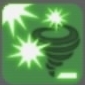Wind Cutter  Fires a small gust of wind at an enemy.

• MP Cost:
• Lv1 & over = 65MP
• Lv200 & over = 80MP
• Lv300 & over = 120MP
• Number of Shots:
• Lv1 = 5
• Lv500 = 18
• Lv1000 = 30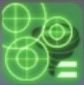Tornado Cannon  Summons multiple whirlwinds upon an enemy.

• MP Cost:
• Lv1 & over = 250MP
• Lv200 & over = 300MP
• Lv300 & over = 400MP
• Number of Shots:
• Lv1 = 3
• Lv500 = 6
• Lv1000 = 9Tyrant Hurricane  Summon massive Hurricane to attack area surrounding target.

• MP Cost:
• Lv1 & over = 1800MP
• Lv200 & over = 2200MP
• Lv300 & over = 3200MPResist Aero  Increases Party Wind Resistance by 33%.

• MP Cost:
• Lv1 & over = 200MP
• Lv200 & over = 220MP
• LV300 & over = 250MP
• Duration:
• Lv1 = 60 Seconds
• LV500 = 90 Seconds
• LV1000 = 180 SecondsEnhance Aero  Increases Party Wind Based Damage by 30%.

• MP Cost:
• Lv1 & over = 300MP
• Lv200 & over = 350MP
• Lv300 & over = 400MP
• Duration:
• Lv1 = 60 Seconds
• Lv500 = 90 Seconds
• Lv1000 = 180 Seconds

### ~EARTH~

#### SKILL DESCRIPTION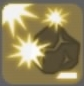Sling Stone  Fires small rocks at an enemy.

• MP Cost:
• Lv1 & over = 5MP
• Lv200 & over = 65MP
• Lv300 & over = 95MP
• Number of Shots:
• Lv1 = 5
• Lv500 = 18
• Lv1000 = 30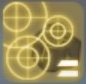Rock Blast  Drops boulders upon an enemy.

• MP Cost:
• Lv1 & over = 600MP
• Lv200 & over = 120MP
• Lv300 & over = 2600MP
• Number of Shots:
• Lv1 = 5
• Lv500 = 18
• Lv1000 = 30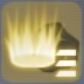Deathrock Cluster  Summons landslide of large rocks drop on an area surrounding target.

• MP Cost:
• Lv1 & over = 1300MP
• Lv200 & over = 1600MP
• Lv300 & over = 2400MPResist Soil  Increases Party Earth Resistance by 33%.

• MP Cost:
• Lv1 & over = 200MP
• Lv200 & over = 220MP
• LV300 & over = 250MP
• Duration:
• Lv1 = 60 Seconds
• LV500 = 90 Seconds
• LV1000 = 180 SecondsEnhance Soil  Increases Party Earth Based Damage by 30%.

• MP Cost:
• Lv1 & over = 300MP
• Lv200 & over = 350MP
• Lv300 & over = 400MP
• Duration:
• Lv1 = 60 Seconds
• Lv500 = 90 Seconds
• Lv1000 = 180 Seconds

### ~DARK~

#### SKILL DESCRIPTIONDark Sphere  Fires a small ball of darkness at an enemy.

• MP Cost:
• Lv1 & over = 60MP
• Lv200 & over = 70MP
• Lv300 & over = 100MP
• Number of Shots:
• Lv1 = 5
• Lv500 = 18
• Lv1000 = 30Void Distortion  Drops large dark clusters upon an enemy.

• MP Cost:
• Lv1 & over = 250MP
• Lv200 & over = 300MP
• Lv300 & over = 400MP
• Number of Shots:
• Lv1 = 3
• Lv500 = 6
• Lv1000 = 9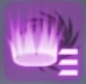Dimensional Abyss  Summon a black hole upon a target to attack surrounding areas.

• MP Cost:
• Lv1 & over = 1600MP
• Lv200 & over = 1850MP
• Lv300 & over = 2500MPResist Dark  Increases Party Dark Resistance by 33%.

• MP Cost:
• Lv1 & over = 200MP
• Lv200 & over = 220MP
• LV300 & over = 250MP
• Duration:
• Lv1 = 60 Seconds
• LV500 = 90 Seconds
• LV1000 = 180 SecondsEnhance Dark  Increases Party Dark Based Damage by 30%.

• MP Cost:
• Lv1 & over = 300MP
• Lv200 & over = 350MP
• Lv300 & over = 400MP
• Duration:
• Lv1 = 60 Seconds
• Lv500 = 90 Seconds
• Lv1000 = 180 Seconds

### ~HOLY~

#### SKILL DESCRIPTIONLightning Arrow  Fires multiple spears of light at an enemy.

• MP Cost:
• Lv1 & over = 58MP
• Lv200 & over = 68MP
• Lv300 & over = 98MP
• Number of Shots:
• Lv1 = 5
• Lv500 = 18
• Lv1000 = 30Holy Lance  Fires a beam of light to pierce an enemy.

• MP Cost:
• Lv1 & over = 650MP
• Lv200 & over = 1350MP
• Lv300 & over = 3100MPDivine Ray  Calls down a ray of light from the heavens to damage an area surrounding a target.

• MP Cost:
• Lv1 & over = 1450MP
• Lv200 & over = 1750MP
• Lv300 & over = 2450MPResist Holy  Increases Party Holy Resistance by 33%.

• MP Cost:
• Lv1 & over = 200MP
• Lv200 & over = 220MP
• LV300 & over = 250MP
• Duration:
• Lv1 = 60 Seconds
• LV500 = 90 Seconds
• LV1000 = 180 SecondsEnhance Holy  Increases Party Holy Based Damage by 30%.

• MP Cost:
• Lv1 & over = 300MP
• Lv200 & over = 350MP
• Lv300 & over = 400MP
• Duration:
• Lv1 = 60 Seconds
• Lv500 = 90 Seconds
• Lv1000 = 180 Seconds

### ~SONG~

#### SKILL DESCRIPTIONSong of Battle  Increases Party Attack by 20%.

• MP Cost: 250MP
• Duration:
• Lv1 = 20 Seconds
• Lv500 = 25 Seconds
• Lv1000 = 30 Seconds
• Range:
• Lv1 = 10m
• Lv500 = 20m
• Lv1000 = 30mSong of Protection  Increases Party Defense by 23%.

• MP Cost: 250MP
• Duration:
• Lv1 = 20 Seconds
• Lv500 = 25 Seconds
• Lv1000 = 30 Seconds
• Range:
• Lv1 = 10m
• Lv500 = 20m
• Lv1000 = 30mSong of Healing  Grants Party HP/MP regeneration.

• MP Cost: 250MP
• Duration:
• Lv1 = 20 Seconds
• Lv500 = 25 Seconds
• Lv1000 = 30 Seconds
• Range:
• Lv1 = 10m
• Lv500 = 20m
• Lv1000 = 30mDivine Prayer  Halves Party’s Stamina Cost.

• MP Cost: 250MP
• Duration:
• Lv1 = 20 Seconds
• Lv500 = 25 Seconds
• Lv1000 = 30 Seconds
• Range:
• Lv1 = 10m
• Lv500 = 20m
• Lv1000 = 30m

### ~FAMILIAR~

#### SKILL DESCRIPTIONFire Breath  Commands familiar to attack target dealing Fire based damage. Power & Range increases with skill level

• MP: 300Ice Breath  Commands familiar to attack target dealing Ice based damage. Power & Range increases with skill level

• MP: 300Wind Breath  Commands familiar to attack target dealing Wind based damage. Power & Range increases with skill level

• MP: 300Sand Breath  Commands familiar to attack target dealing Earth based damage. Power & Range increases with skill level

• MP: 300Shadow Breath  Commands familiar to attack target dealing Dark based damage. Power & Range increases with skill level

• MP: 300Lightning Breath  Commands familiar to attack target dealing Holy based damage. Power & Range increases with skill level

• MP: 300Heal Breath  Commands familiar to heal self, recovering HP. Party members standing nearby can also be healed.

• MP: 400
• Recovery Amount:
• Lv0 = 15%
• Lv500 = 28%
• Lv1000 = 40%Cure Breath  Commands familiar to heal self, curing bad statuses.

• MP: 400
• Number of Ailments Recovered:
• Lv0 = 1
• Lv500 = 2
• Lv1000 = 4Silver Headbutt  Pina’s OSS. Inspired by Silver Crow & Agil. Pina rushes at the target giving it a hard headbutt.

• MP: 300

———————————–